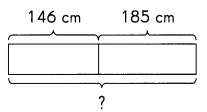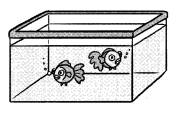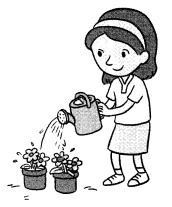# Math in Focus Grade 3 Chapter 12 Practice 1 Answer Key Real-World Problems: One-Step Problems

Practice the problems of Math in Focus Grade 3 Workbook Answer Key Chapter 12 Practice 1 Real-World Problems: One-Step Problems to score better marks in the exam.

## Math in Focus Grade 3 Chapter 12 Practice 1 Answer Key Real-World Problems: One-Step Problems

Question 1.
A restaurant has two tables of different lengths. One is 146 centimeters long. The other is 185 centimeters long. What is their total length? Write your answer in meters and centimeters.There total length is 331 centimeters
There total length is 3.31 meters
Explanation:
A restaurant has two tables of different lengths. One is 146 centimeters long.
The other is 185 centimeters long.
145 + 185 = 331 cm
3.31 meters
1 meter = 100 centimeters

Question 2.
Jim is preparing for a race. He runs around the track 3 times each day. If he runs 300 meters a day, what is the length of the track?
100 meters
Explanation:
Jim is preparing for a race. He runs around the track 3 times each day.
If he runs 300 meters a day,
300 ÷ 3 = 100 meters is the length of the track

Question 3.
A baby elephant has a mass of 1 45 kilograms. The mass of its sister is 5 times as much. What is the mass of its sister?
The mass of its sister = 725 kilograms
Explanation:
A baby elephant has a mass of 1 45 kilograms. The mass of its sister is 5 times as much.
145 x 5 = 725

Question 4.
James has a fish tank with a capacity of 24 liters. He uses a 2-liter pail to fill the tank. How many pails of water does he use?12 pails of water that he use
Explanation:
James has a fish tank with a capacity of 24 liters.
He uses a 2-liter pail to fill the tank.
24 ÷  2 = 12

Question 5.
A watering can contains 980 milliliters of water. Some water is used to water the plants. In the end, 350 milliliters of water are left. How much water is used to water the plants?630 milliliters water is used to water the plants
Explanation:
A watering can contains 980 milliliters of water.
Some water is used to water the plants.
In the end, 350 milliliters of water are left.
980 – 350 = 630

Question 6.
A baker has 2,000 grams of flour. He uses 425 grams of it. What is the mass of the flour left? Give your answer in kilograms and grams.
In kilograms = 1.575
In grams = 1575
Explanation:
A baker has 2,000 grams of flour. He uses 425 grams of it.
2000 – 425 = 1575
1 kg = 1000 grams
1.575 grams

Question 7.
Max buys 875 grams of nuts on Monday. He buys 955 grams of nuts on Tuesday. What is the total mass of nuts he buys? Give your answer in kilograms and grams.
the total mass of nuts he buys = 1830 grams
In kilograms = 1.830 Kilograms.
Explanation:
Max buys 875 grams of nuts on Monday. He buys 955 grams of nuts on Tuesday.
875 + 955 = 1830 grams

Question 8.
Mrs. Spence has 4 bottles of orange juice. Each bottle contains 125 milliliters of juice. She pours all the juice into an empty container. How much orange juice is in the container?